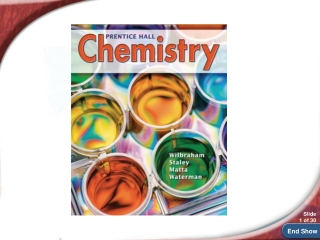DownloadDownload PresentationChemistry 14.4

# Chemistry 14.4

Télécharger la présentation## Chemistry 14.4

- - - - - - - - - - - - - - - - - - - - - - - - - - - E N D - - - - - - - - - - - - - - - - - - - - - - - - - - -
##### Presentation Transcript

1. Chemistry 14.4

2. Ideal Gases and Real Gases • Under what conditions are real gases • most likely to differ from ideal gases? • Ideal gases obey the gas laws and behave according to the Kinetic Molecular Theory. No gas is a true ideal gas but He and H2 behave most like an ideal gas especially when P is low and T is high. • There are attractions between the particles in a gas. Because of these attractions, a gas can condense,or even solidify, when it is compressed or cooled.

3. Real gases differ most from an ideal gas at low temperatures and high pressures. • Gases behave most like an ideal gas at high temperatures and low pressures.

4. Gases: Mixtures and Movements • A list of gear for an expedition to Mount Everest includes climbing equipment, ski goggles, a down parka with a hood, and most importantly compressed-gas cylinders of oxygen. You will find out why a supply of oxygen is essential at higher altitudes.

5. Dalton’s Law • How is the total pressure of a mixture of gases related to the partial pressures of the component gases? • Dalton’s law of partial pressures states that, at constant volume and temperature, the total pressure exerted by a mixture of gases is equal to the sum of the partial pressures of the component gases.

6. Dalton’s Law • The contribution each gas in a mixture makes to the total pressure is called the partial pressure exerted by that gas.

7. Dalton’s Law • Three gases are combined in container T.

8. Dalton’s Law • The partial pressure of oxygen must be 10.67 kPa or higher to support respiration in humans. The climber below needs an oxygen mask and a cylinder of compressed oxygen to survive.

9. 14.6

10. 14.6

11. 14.6

12. for Sample Problem 14.6 • INDEX CARD activity (turn in for evaluation)

13. Graham’s Law • How does the molar mass of a gas affect the rate at which the gas effuses or diffuses? • Diffusion is the tendency of molecules to move toward areas of lower concentration until the concentration is uniform throughout. During effusion, a gas escapes through a tiny hole in its container.

14. Graham’s Law • Gases of lower molar mass diffuse and effuse faster than gases of higher molar mass. • Thomas Graham’s Contribution: Graham’s law of effusion states that the rate of effusion of a gas is inversely proportional to the square root of the gas’s molar mass. This law can also be applied to the diffusion of gases.

15. Graham’s Law • Comparing Effusion Rates • A helium filled balloon will deflate sooner than an air-filled balloon. • Helium atoms are less massive than oxygen or nitrogen molecules. So the molecules in air move more slowly than helium atoms with the same kinetic energy.

16. Graham’s Law • Thomas Graham’s Contribution • Graham’slaw of effusion states that the rate of effusion of a gas is inversely proportional to the square root of the gas’s molar mass. This law can also be applied to the diffusion of gases.

17. Graham’s Law • Helium effuses (and diffuses) nearly three times faster than nitrogen at the same temperature. • Animations and practice problems for diffusion and effusion

18. 14.4 Section Quiz. • 14.4.

19. 14.4 Section Quiz. • 1. What is the partial pressure of oxygen in a diving tank containing oxygen and helium if the total pressure is 800 kPa and the partial pressure of helium is 600 kPa? • 200 kPa • 0.75 kPa • 1.40 104 kPa • 1.33 kPa

20. 14.4 Section Quiz. • 2. A mixture of three gases exerts a pressure of 448 kPa, and the gases are present in the mole ratio 1 : 2 : 5. What are the individual gas pressures? • 44 kPa, 88 kPa, and 316 kPa • 52 kPa, 104 kPa, and 292 kPa • 56 kPa, 112 kPa, and 280 kPa • 84 kPa, 168 kPa, and 196 kPa

21. 14.4 Section Quiz. • 3. Choose the correct words for the spaces. Graham's Law says that the rate of diffusion of a gas is __________ proportional to the square root of its _________ mass. • directly, atomic • inversely, atomic • inversely, molar • directly, molar